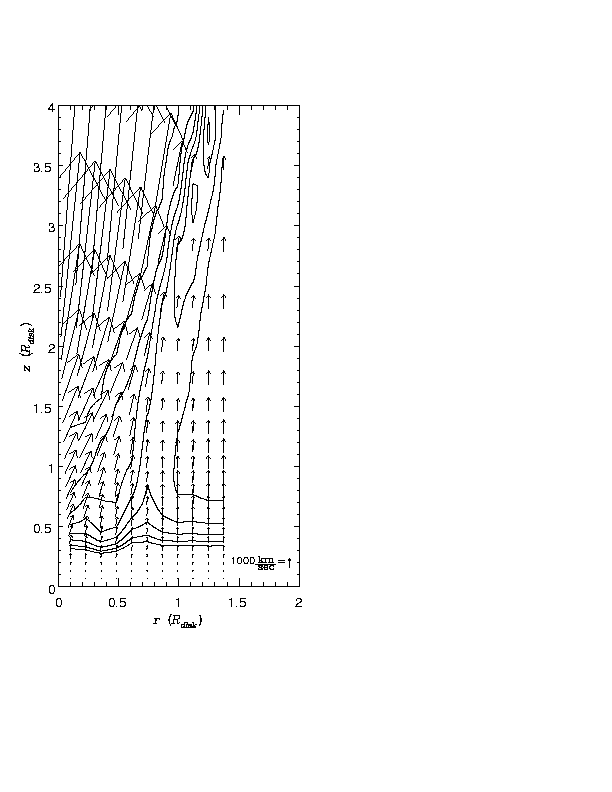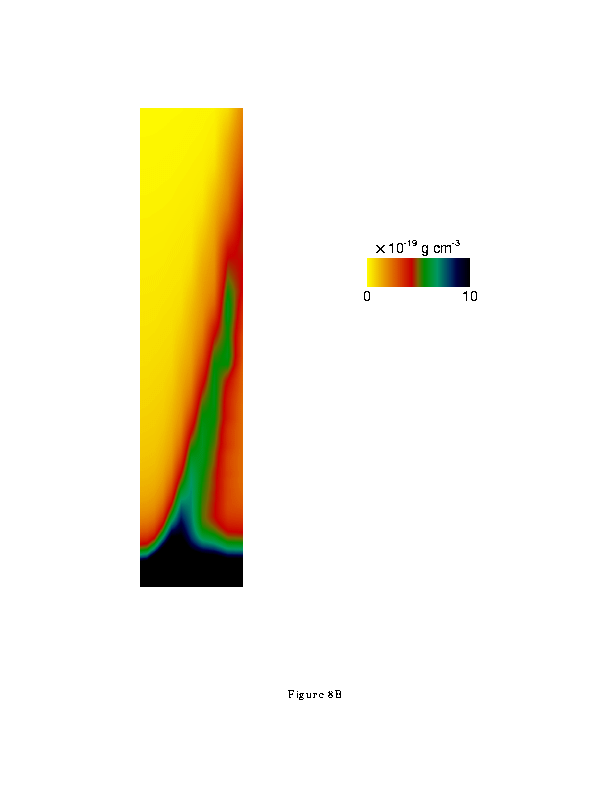[an error occurred while processing this directive]

Accretion Disk Winds

## Accretion Disk Winds

### Background

The existence of winds from cataclysmic variables (CVs) has been known for some time, there are a variety of unresolved questions surrounding this phenomenon. These include: the origin of the apparent polar nature of the winds; the rate of mass loss in the winds and the associated driving mechanism; the origin for the characteristic shapes of the UV resonance line profiles, particularly the absorption component; and the apparent association between outburst state and the wind existence. Resolution of all these issues depends on understanding the dynamics of the wind. We have developed one and two dimensional hydrodynamical models for these winds which explains many of the observed properties

### One Dimensional Models

Our procedures for treating the winds in one dimension are analagous to those developed for radiation pressure driven winds from stars by, for example, Castro Abbott and Klein (1978; CAK). However, the critical point conditions in the disk case are more complicated owing to the fact that in the disk wind problem the force of gravity per mass along the stream lines increases. Also there is no spherical symmetry in the disk wind, thus we introduce a geometric correction for the mass conservation equation which reflects the fact that the wind streamlines are likely to start out vertically relative to the disk plane, and end up more nearly radial relative to the central star far from the disk. As a result, where CAK obtained an analytic expression for the mass loss rate in the wind and for the velocity law far from the critical point, we are forced to solve our transcendental equation numerically, and cannot present simple analytic expressions for the corresponding quantities.

Additional wind solutions are obtained by varying L_disk from 0.1 L_sun to 10 L_sun , for a value of R_disk=R_sun . We find that the terminal speed is approximately independent of luminosity. Early type stars with line driven winds also show terminal speeds approximately independent of luminosity (citecas75,abb78). We also found that mass loss rates depend on the luminosity of the disk according to L_disk^+1.4288 approximately, again analogous to line driven winds of early type stars which show a dependence of L_star^1/ alpha (in our models 1 over alpha=1 over 0.7 approx +1.4286 ).

From our one dimensional models we are able to identify a criterion for the existence of winds in terms of the logarithmic derivitive of the radiation field with height above the disk. That is, if this quantity does not increase with height faster than z^{1/2}, there can be no wind solution. In other words, a minimum of optical depth of the lines driving the wind is necessary for a wind to exist. This contrasts with the case of early type stars where a wind will always develop for the values of alpha between zero and one. The minimum optical depth that we find to be a requirement for the existence of disk winds may be physically interpreted as the necessity of the wind to surpass the barrier of an increasing gravity, which is a peculiarity of the disk wind case.

### Two Dimensional Models

The gas flow near a disk is inherently two dimensional, and one dimensional models require severe approximations which may limit their validity. Therefore, we have constructed a two dimensional numerical model for line driven winds near CV disks. The numerical method we adopt is based on the PPM (Piecewise Parabolic Method) numerical scheme. The PPM method has been discussed in detail by Colella and Woodward (1984) for a one dimensional spatial grid with planar, cylindrical or spherical symmetries.

The most important feature of the 2D model results, the role of rotational forces, is apparent in the figure. Gas leaving the disk is accelerated upwards by the radiation pressure force. However, at a height comparable to the disk radius the Keplerian balance, which initially allows a stable flow in the direction normal to the disk, is lost. The wind flows radially above this region. This effect occurs at all radii, but the strength of the radial force is greater at smaller radii. Thus the initially vertical streamlines are bent most at small radius, with the result that they collide with more nearly vertical streamlines of material originating at large radii. This collision results in shocks and a large region of enhanced density which runs diagonally in the z - r plane.Figure 1a: Contours of constant density superimposed on velocity vectors as a function of position from a simulation of a line driven disk wind. Position is expressed in units of the fiducial radius, chosen to be one solar radius.Figure 1b Color contour plot of density for the same simulation shown in figure 1a. Density scale is shown in the upper right.

[an error occurred while processing this directive]

[an error occurred while processing this directive]